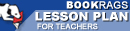Lesson Plans

# The Logic of Scientific Discovery Test | Final Test - Medium

This set of Lesson Plans consists of approximately 102 pages of tests, essay questions, lessons, and other teaching materials.
 View a FREE sampleName: _________________________ Period: ___________________

This test consists of 5 multiple choice questions, 5 short answer questions, and 10 short essay questions.

## Multiple Choice Questions

1. What does Popper feel about normally attained simplicity?
(a) It is untenable.
(b) It is logical.
(c) It is too subjective.
(d) It serves to provide a justification.

2. What concept does Popper consider probability to be outside of?
(a) Positivism.
(b) Falsification.
(c) Conventionalism.
(d) Simplicity.

3. What is the result, according to Popper, when inductive logic is used?
(a) Finite regress.
(b) Infinite regress.
(c) Statistical significance.
(d) Falsifiable theories.

4. What is retained by old theories when their success is demonstrated through research?
(a) Regularities.
(b) Assumptions.
(c) Validity.
(d) Determinants.

5. What is able to refute portions of old theories?
(a) Quantum physics.
(b) Logic.
(c) New theories.
(d) New experiments.

## Short Answer Questions

1. According to Popper, since measurement can be altered what does that say about using measurement in science?

2. What hypothetical estimate of frequency suggests equal distribution, normally based on considerations of symmetry?

3. What does Popper eliminate from the common frequency theory by using mathematical and narrative approaches?

4. What does Popper state cannot be applied to a sequence of events?

5. By seeking the highest degree of universality and precision, what type of content results?

## Short Essay Questions

1. What are the two main sources for hypothetical estimates of frequencies with regard to infinite empirical sequences?

2. According to Popper, where does the value of simplicity lay?

3. How is the theory of chance solved?

4. Using Heisenberg's Uncertainty Principle, what does Popper attempt to prove?

5. What two scientific arguments does Popper use to verify his beliefs about inductivists?

6. What is the basis of the wave theory used by Schrödinger?

7. Why does Popper feel the view of Wittgenstein, Schlick, and Feigl regarding simplicity misses crucial points?

8. What does Popper believe regarding the logical proximity of a theory to empirical statements?

9. How is the physical basis for the uncertainty theory undermined?

10. How does Popper feel traditional inductivists work?

(see the answer keys)

 This section contains 781 words (approx. 3 pages at 300 words per page) View a FREE sampleMore summaries and resources for teaching or studying The Logic of Scientific Discovery.